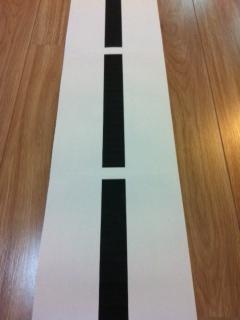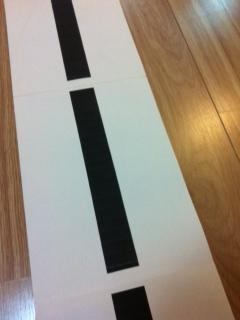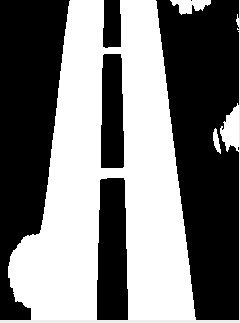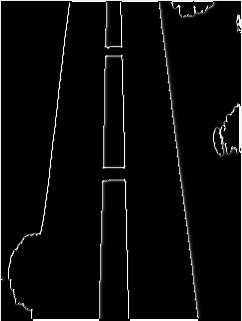2 views

I'm processing some images that my UGV (Unmanned Ground Vehicle) captures to make it move on a line.

I want to get the angle of that line based on the horizon. I'll try to explain with a few examples:The image above would make my UGV to keep straight ahead, as the angle is about 90 degrees. But the following would make it turn left, as the angle compared to the horizon rounds about 120.I could successfully transform those images into the image below using Otsu for thresholding:And also used an edge detection algorithm to get this:But I'm stuck right now trying to find an algorithm that detects those edges/lines and outputs - or helps me to output - the angle of such line...

by (108k points)

While the edges of the image(boundaries between the regions with relatively distinct gray levels) are the most common type of discontinuity of an image, instances of thin lines in an image occur frequently enough that it is useful to have a separate mechanism for detecting the lines. There is a convolution-based technique that produces an image description of the thin lines in an input image. Note that the Hough transform can be used to detect lines; however, in that case, the output is a parametric description of the lines in an image.

For a better understanding of the Hough transform, refer the following link:

You can refer the following link for the algorithm:

https://raw.githubusercontent.com/opencv/opencv/master/samples/java/tutorial_code/ImgTrans/HoughLine/HoughLines.java

If you wish to know about Line detection and Angle detection with java then visit this Java Tutorial.